Next: Properties of Spin Angular Up: Spin Angular Momentum Previous: Spin Angular Momentum

# Introduction

Up to now, we have tacitly assumed that the state of a particle in quantum mechanics can be completely specified by giving the wavefunctionas a function of the spatial coordinates,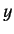, and. Unfortunately, there is a wealth of experimental evidence that suggests that this simplistic approach is incomplete.

Consider an isolated system at rest, and let the eigenvalue of its total angular momentum be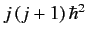. According to the theory of orbital angular momentum outlined in Sections 4.4 and 4.5, there are two possibilities. For a system consisting of a single particle,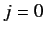. For a system consisting of two (or more) particles,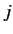is a non-negative integer. However, this does not agree with observations, because we often encounter systems that appear to be structureless, and yet have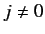. Even worse, systems wherehas half-integer values abound in nature. In order to explain this apparent discrepancy between theory and experiments, Gouldsmit and Uhlenbeck (in 1925) introduced the concept of an internal, purely quantum mechanical, angular momentum called spin. For a particle with spin, the total angular momentum in the rest frame is non-vanishing.Next: Properties of Spin Angular Up: Spin Angular Momentum Previous: Spin Angular Momentum
Richard Fitzpatrick 2013-04-08